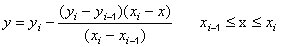Michael Thomas Flanagan's Java Scientific LibraryLinearInterpolation Class:     Linear Interpolation

Last update: 24 February 2011                                                                                                                              Main Page of Michael Thomas Flanagan's Java Scientific Library

This class contains the constructor and methods for performing an interpolation within a one dimensional array of data points, y = f(x), using a linear interpolation:import directive: import flanagan.interpolation.LinearInterpolation;
 Constructor public LinearInterpolation(double[] x, double[] y) Interpolate public double interpolate(double xx) Rounding Error Options public static void noRoundingErrorCheck() public static void potentialRoundingError(double potentialRoundingError)

CONSTRUCTOR

CONSTRUCTOR
public LinearInterpolation(double[] x, double[] y)
Usage:                      LinearInterpolation aa = new LinearInterpolation(x, y);
Creates an instance of LinearInterpolation with its internal data arrays initialised to copies of the values in the x and y where y is the tabulated function y = f(x).

METHODS

INTERPOLATION
public double interpolate(double xx)
Usage:                      y1 = aa.interpolate(xx1);
Returns the interpolated value of y, y1, for a given value of xx1, using the y = f(x) data entered via the constructor. This method may be called as often as required. This method throws an IllegalArgumentException if xx1 is outside the range of the values of x[] supplied to the constructor.

ROUNDING ERROR OPTIONS
public static void noRoundingErrorCheck()
Usage:                      LinearInterpolation.noRoundingErrorCheck();
Seveveral applications that call LinearInterpolation, e.g. plotting programs, may calculate an array of points to be fed to an instance of linearInterpolation that should lie between the limits initially supplied to the instance but which, due to rounding errors in the calculation of the array, may have extreme values that lie outside the limits by the an amount equal to the rounding error, e.g. an array that should lie between limits of 0 and 4 may run from 0 to 4.0000000000000003. The default option of the LinearInterpolation class is to check for such violations of the order of a rounding error and round the extreme value to the limit thus preventing an out of range exception being thrown. This method allows this default option to be ignored so that an out of range exception is thrown if any value lies outside he range of the limis no matter how small the violation is.

public static void potentialRoundingError(double potentialRoundingError)
Usage:                      LinearInterpolation.potentialRoundingError(potentialRoundingError);
Seveveral applications that call LinearInterpolation, e.g. plotting programs, may calculate an array of points to be fed to an instance of LinearInterpolation that should lie between the limits initially supplied to the instance but which, due to rounding errors in the calculation of the array, may have extreme values that lie outside the limits by the an amount equal to the rounding error, e.g. an array that should lie between limits of 0 and 4 may run from 0 to 4.0000000000000003. The default option of the LinearInterpolation class is to check for such violations of the order of a rounding error and round the extreme value to the limit thus preventing an out of range exception being thrown. The default calculculation of the potential rounding error is the multiplication of 5x10-15 by 10 raised to the exponent of the value lying outside the limits. This method allows the value of 5x10-15 to be reset to the user's choice, potentialRoundingError.

OTHER CLASSES USED BY THIS CLASS

This class uses the following classes in this library: